# Gradient descent with constant learning rate for a quadratic function of one variable

## Setup

### Function

Explicitly, we have a quadratic function:$f(x) := ax^2 + bx + c$

where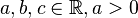$a,b,c \in \R, a > 0$.

Recall that the unique point of local minimum, that is also the point of absolute minimum, is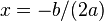$x = -b/(2a)$. The value of the minimum is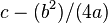$c - (b^2)/(4a)$.

Quadratic functions of one variable have a nice property: the value of the quadratic function at a point is an increasing function of its distance from the point of local minimum. Therefore, getting a closer point in the domain is equivalent to getting a smaller function value, and we do not need to keep track of the distinction.

Recall also that the derivatives are given by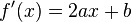$f'(x) = 2ax + b$ and$f''(x) = 2a$.

### Learning algorithm

Suppose$\alpha$ is a positive real number. The gradient descent with constant learning rate$\alpha$ is an iterative algorithm that aims to find the point of local minimum for$f$. The algorithm starts with a guess$x^{(0)}$ and updates according to the rule: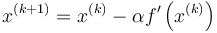$x^{(k+1)} = x^{(k)} - \alpha f'\left(x^{(k)}\right)$

Note that we use parenthesized superscripts to denote the iterates, and these should not be confused with exponents. The reason for using superscripts instead of subscripts is to keep notation consistent with the case of functions of multiple variables, where subscripts are used for coordinates and superscripts for iterates.

## Closed form expressions for iterates

Recall the above expression:$x^{(k+1)} = x^{(k)} - \alpha f'\left(x^{(k)}\right)$

Plugging in values, we obtain:$x^{(k+1)} = x^{(k)} - \alpha \left(2ax^{(k)} + b\right)$

This is neater if we subtract the optimum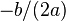$-b/(2a)$ from both sides:$x^{(k+1)} - \frac{-b}{2a} = x^{(k)} + \frac{b}{2a} - 2a\alpha \left(x^{(k)} - \frac{-b}{2a}\right)$

We can now rewrite as: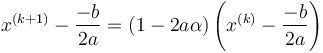$x^{(k+1)} - \frac{-b}{2a} = (1 - 2a\alpha) \left(x^{(k)} - \frac{-b}{2a}\right)$

In other words, the difference with$-b/(2a)$ multiplies by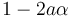$1 - 2a\alpha$ in each iteration. We therefore obtain this closed-form expression for the iterates:$x^{(k)} = \frac{-b}{2a} + (1 - 2a\alpha)^k \left(x^{(0)} - \left( \frac{-b}{2a}\right)\right)$

## Convergence properties based on the learning rate

### Summary

Assume that the initial point for the gradient descent is not already the point of minimum$x = -b/(2a)$. Then, the sequence converges if and only if:$|1 - 2a\alpha| < 1$

This is equivalent to the condition:$\left| \alpha - \frac{1}{2a}\right| < \frac{1}{2a}$

or equivalently, that: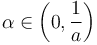$\alpha \in \left(0, \frac{1}{a}\right)$

Moreover, if so, the convergence is linear convergence with convergence rate:$|1 - 2a\alpha| = \left|1 - \frac{\alpha}{1/(2a)}\right|$

### Details

As before, we assume that the initial guess is not already the point of minimum.

Case on$\alpha$ Does the sequence converge to the minimum? How does it behave? Convergence rate$\alpha = 0$ No. The sequence stays stuck at the initial point. --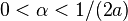$0 < \alpha < 1/(2a)$ Yes. The sequence converges monotonically to the minimum. The convergence is linear convergence and the convergence rate is$1 - 2a\alpha = 1 - \frac{\alpha}{1/(2a)}$. The closer$\alpha$ is to$\frac{1}{2a}$, the faster the convergence. Note that the convergence rate is the same at every step rather than simply being a limiting value.$\alpha = 1/(2a)$ Yes. Convergence happens in one step. Since convergence occurs in one step, it does not make sense to talk of the convergence rate.$1/(2a) < \alpha < 1/a$ Yes. The convergence is oscillatory (the sequence switches sides with respect to the minimum each time), but the value gets closer to the root in each step. The convergence is linear convergence and the convergence rate is$2a\alpha - 1 = \frac{\alpha}{1/(2a)} - 1$. The closer$\alpha$ is to$\frac{1}{2a}$, the faster the convergence. Note that the convergence rate is the same at every step rather than simply being a limiting value.$\alpha = 1/a$ No. The sequence oscillates between the points$x_0$ and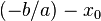$(-b/a) - x_0$, both equidistant from the point of minimum and on opposite sides of it. --$\alpha > 1/a$ No. The sequence oscillates around the root (each time, it switches side with respect to the minimum) and each member is further from the minimum than its predecessor. --